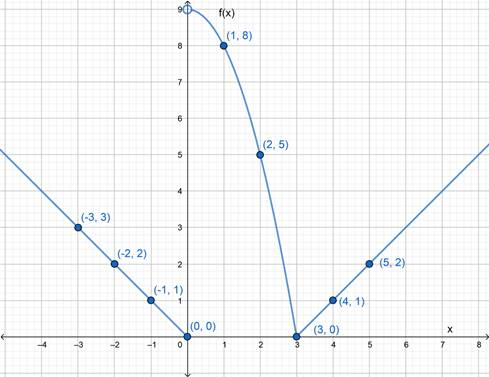# The graph of the given function.### Precalculus: Mathematics for Calcu...

6th Edition
Stewart + 5 others
Publisher: Cengage Learning
ISBN: 9780840068071### Precalculus: Mathematics for Calcu...

6th Edition
Stewart + 5 others
Publisher: Cengage Learning
ISBN: 9780840068071

#### Solutions

Chapter 2.2, Problem 46E
To determine

## To sketch : The graph of the given function.

Expert Solution

### Explanation of Solution

Given information :

f(x)={x             if  x09x2        if 0<x3 x3          if x>3

Graph :

 x −3 −2 −1 0 1 2 3 4 5 f(x) 3 2 1 0 8 5 0 1 2This is the graph of the given piecewise defined equation.

Interpretation : The part of the graph to the left of x=0 coincides with the graph of f(x)=x and the part of the graph to the right of x=3 coincides with the graph of f(x)=x3 . The part of the graph between x=0 and x=3 coincides with f(x)=9x2 .

### Have a homework question?

Subscribe to bartleby learn! Ask subject matter experts 30 homework questions each month. Plus, you’ll have access to millions of step-by-step textbook answers!